04 Jul
04Jul

## General Definition:

As I think everybody learnt about this algorithm in high school, I will just revise the definition of it here and see what data science tries to achieve with it.

It is a linear approach for modelling the relationship between a scalar dependent variable y and one or more explanatory [Independent] variables denoted X.

So, in data science, our goal is to find answer for - Is the variable X (or more likely, X1,.....XP) associated with a variable Y?, and, if so, what is the relationship and can we use it to predict Y?

## Types:

Linear regression algorithm has two types:

• #### Simple Linear Regression

[The case of one explanatory (independent) variable is called simple linear regression]

• #### Multiple Linear Regression

[The case of more than one explanatory (independent) variables is called multiple linear regression]

When we are talking about relation of one variable to other, it can be possible in two ways : Correlation [measures the strength of an association between two variables] or Regression [quantifies the nature of the relationship]

## Yi = b0 + b1Xi + ei

[Yi equals b1 times Xi plus constant b0]

As discussed above, Xi is independent variable and Yi is dependent variable. b0 is constant (or intercept), b1 is slope for Xi and ei is Random error value for this Xi.With the correlation coefficient, the variables Xi and Yi are interchangeable. With regression, we are trying to predict the Yi variable from Xusing a linear relationship.

In Data Science terms, Yi is a target and  Xi is a feature vector.

The fitted values, also referred to as the predicted values, are given by: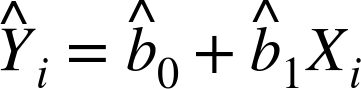The notation b0 and b1 with hat (carot) indicates that the coefficients are estimated versus known.

Now, compute the residuals ei with hat (carot)  by subtracting the predicted values from the original data as below:

##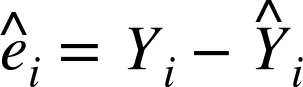The sum of the squared residuals is termed least squares regression, or ordinary least squares (OLS) regression.

The regression line is the estimate that minimizes the sum of squared residual values, also called the residual sum of squares or RSS: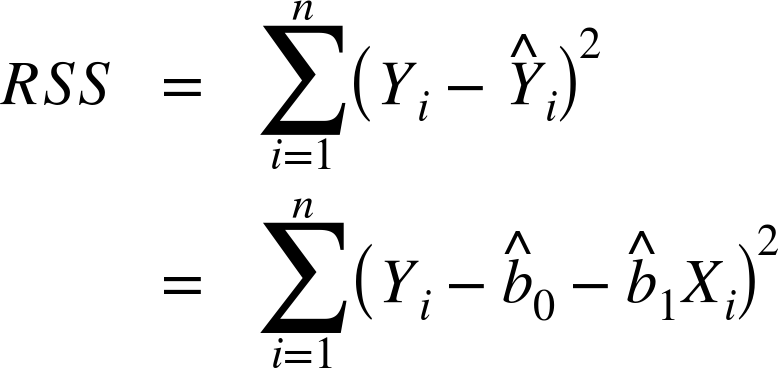Least squares regression leads to a simple formula to compute the coefficients: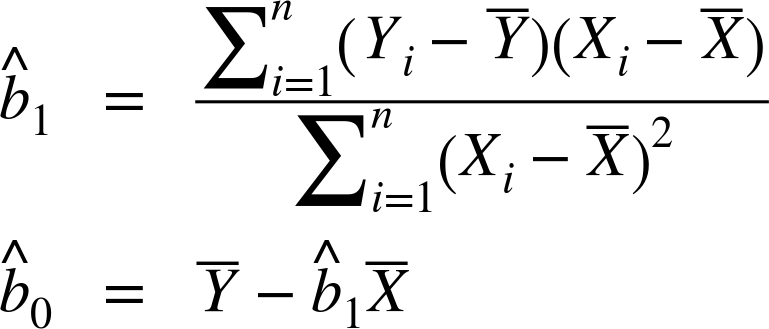With this, there are two more terms, outliers and medians. This I will cover in my GitHub repository code along with example of real dataset.

## Simple Linear Regression Model using R Programming

lm function in R is used for linear regression.

It can be written as below:

#### model <- lm(Yi ~ Xi, data= dataset)

"lm" stands for linear model and "~" symbol denotes that Yi is predicated by Xi.

Running this model produces following output:

Call:

lm(formula = Yi ~ Xi, data= dataset)

Coefficients:

(Intercept)          Exposure

value                value

So values in exposure is negative for positive value of intercept, it can be interpreted as for increase of X the value of Y gets reduced. This correlation I will talk more in my second multilinear regression model.

In approximate, the linear regression looks like this: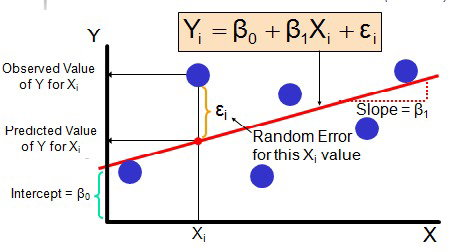After that moving to fitted and residuals values can be calculated  using predict and residuals functions as below:

#### residuals<-residuals(model)

You can refer figure below to see the residuals. So these vertical lines are nothing but the residuals for given linear regression line.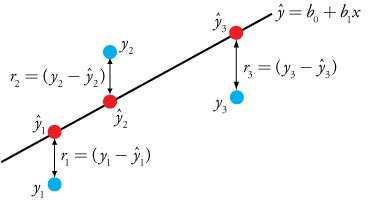## Simple Linear Regression using Python Programming

This is part of my code for Lending Club Loan dataset, to predict the interest rate.

#Import required libraries

import matplotlib.pyplot as plt
import numpy as np
import os

import pandas as pd
from sklearn import linear_model
from sklearn import model_selection
from sklearn import preprocessing

#Create linear regression object
lm = linear_model.LinearRegression()

# Train the model
lm.fit(train_df_x, train_df_y)

# Print the coefficients
print('Coefficients: \n', lm.coef_)

# Calculate the mean squared error
print("Mean squared error: %.2f" % np.mean((lm.predict(test_df_x) - test_df_y) ** 2))
print("Root mean squared error: %.2f" % (np.mean((lm.predict(test_df_x) - test_df_y) ** 2))**0.5)

# Print the explained variance score (1 is perfect prediction)
print('Variance score: %.2f' % lm.score(test_df_x, test_df_y))

print("Mean Absolute Percent Error: %.2f" % (np.mean(np.abs((lm.predict(test_df_x) - test_df_y) / lm.predict(test_df_x))) * 100))

Output:

Coefficients:  [[ 0.46728469  1.70537976 -0.02228413 -0.19746662  0.52047471  0.08683277  -0.19135679  0.83562216 -0.09196297 -0.05546849 -0.24220846  0.3096084  -0.70188735 -0.00500134  0.81432747 -0.08992683 -0.40736942 -0.02532263   0.01828071  0.01658771  0.03755726 -1.20824699 -0.21832669  0.00521397   0.02148635 -0.02096725  0.39450338]]Mean squared error: 11.62Root mean squared error: 3.41Variance score: 0.45Mean Absolute Percent Error: 21.43

For complete code you can visit my Git Hub repository: https://github.com/vaishalilambe/Lambe_Vaishali_DataScience_Python_2017/blob/master/Assignment2/part2/interest-rate-prediction-linear-regression.ipynb

If you need any clarifications/ have any suggestions/feedback please feel free to comment. I will update it accordingly and take care in next algorithm blog.

I am setting up folder in my GitHub repository for algorithms, you will find more examples of linear regression with R and Python soon. Also I will provide some instructions on how to run it.

Stay tuned....comping up next...Multiple Linear Regression